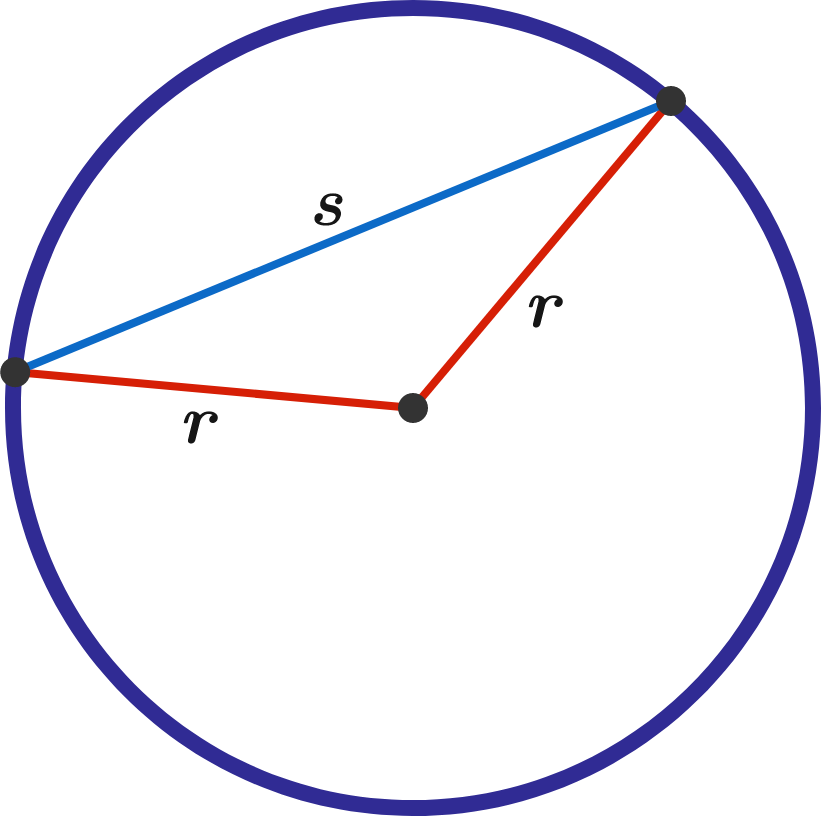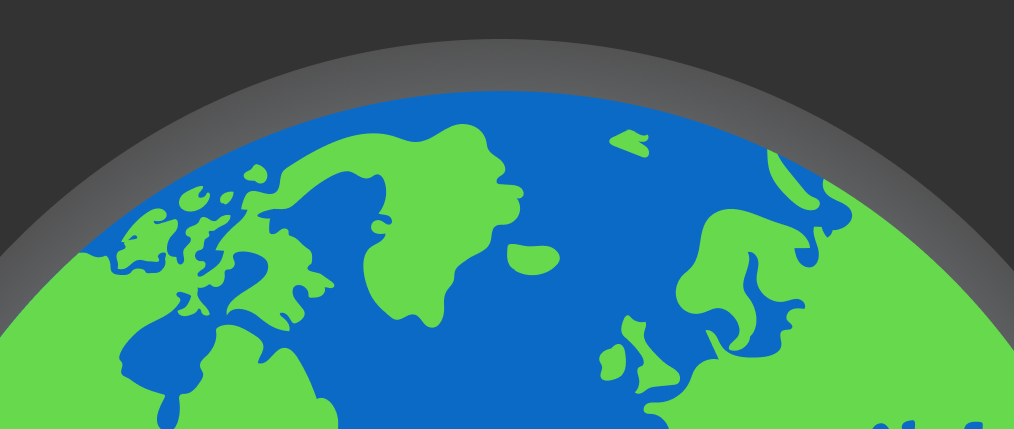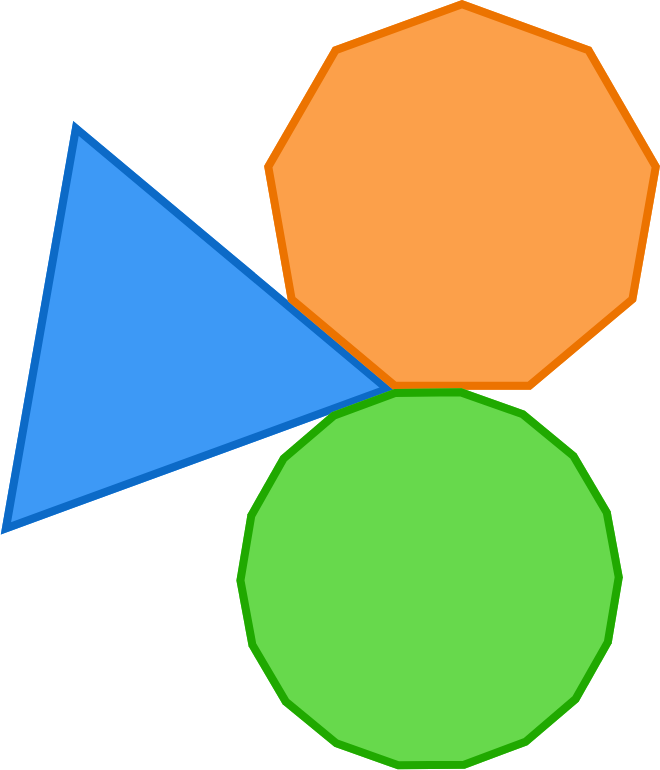# Problems of the Week

Contribute a problem

# 2018-03-05 Intermediate

Two points are placed randomly along the circumference of a circle. What is the probability that the chord drawn between them is longer than the radius of the circle?How often is Length of Chord > Radius?

A positive integer $n$ has $d$ positive divisors.

After doubling $n,$ you find that $2n$ has $2d$ positive divisors.

Is it possible that after tripling $n,$ you will find that $3n$ has $3d$ positive divisors?The atmosphere is a layer of gas that surrounds our planet and is several hundred kilometers thick. Its main components are nitrogen, oxygen, argon, and water vapor.

What is the approximate mass $m$ of the entire Earth's atmosphere?

Details and Assumptions:

• The circumference of the Earth is about $40,000 \text{ km}.$
• Gravitational acceleration is approximately $10 \text{ m/s}^2.$
• The mean air pressure on the Earth's surface is about $1 \text{ bar } \big(\SI{1}{bar} = \SI{10^5}{Pa} \big).$

Define the star operation as $a\star b=a+ab+b.$

Then define the star power operation as $a^{\star n}=\underbrace{a\star a\star a\star\cdots\star a}_{a\, \mathrm{written} \, n \, \mathrm{times}},$ with $a^{\star 1}=a.$

What is $7^{\star7}?$

An equilateral triangle and 2 other regular polygons share a single vertex in such a way that the three shapes completely cover the $360^\circ$ of space surrounding the vertex without overlapping.

What is the largest possible number of sides that one of the regular polygons can have?An equilateral triangle, regular 9-gon, and regular 18-gon can cover all $360^\circ$ around a single vertex. Is 18 the maximum number of sides one of these regular polygons can have?

×